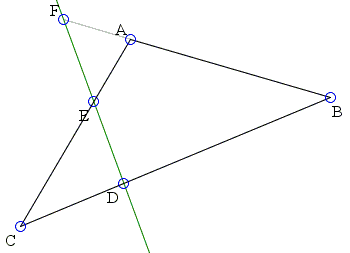# Menelaus from 3D

Invocation of 3D imagery often serves a powerful tool in proving theorems of plane geometry. Farther down the page there are links to several demonstrations of this idea. On November 28, 2010, Hubert Shutrick had a vision that rendered a one-liner proof of Menelaus' theorem.Let three points F, D, and E, lie respectively on the sides AB, BC, and AC of ΔABC or their extensions. Assume just one or all three of the points lie on side extensions. Then the points are collinear iff

 AFBF
·
 BDCD
·
 CEAE
= 1

### Proof (Hubert Shutrick)

Put up a flagpole AA' orthogonal to the plane and let B' and C' be the points where the orthogonal lines through B and C meet the plane A'DEF. If the heights of the verticals are ha, hb and hc, possibly negative, then similar vertical right triangles give

ha/hb = AF/BF, hb/hc = BD/CD and hc/ha = CE/AE

which, when multiplied together, produce exactly the required identity.

Conversely, assume Menelaus' identity holds. Erect AA' and consider the plane A'EF. Define B' and C' as before, and D' the intersection of B'C' with BC. Then

ha/hb = AF/BF, hb/hc = BD'/CD' and hc/ha = CE/AE.

Comparing the product of the three with Menelaus' identity shows that BD'/CD' = BD/CD, implying D = D' and, subsequently, the collinearity of D with E and F.### 2D Problems That Benefit from a 3D Outlook71240284